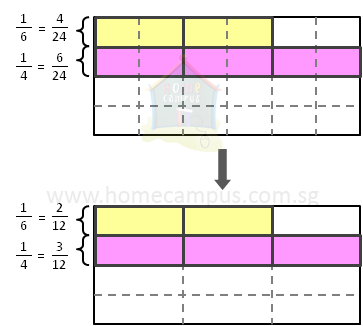## Comparing Unlike Fractions and Least Common Multiple Part 2

### What are like fractions?

Like fractions are two or more fractions that have the same denominator.

Example:

 15 and 25 (both these fractions have the same denominator of 5)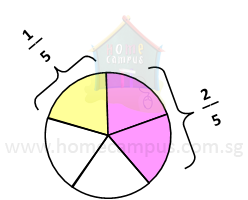### What are unlike fractions?

Unlike fractions are two or more fractions that do not have the same denominator.

Example:

 13 and 14 (the denominators of these two fractions are different)

### How do you compare unlike fractions?

Example:

 Compare 13 and 14 .
 Let's use lasagnas to mark these two fractions. Since the given fractions have unlike denominators, we cannot mark them on a single lasagna. Let's use two lasagnas instead.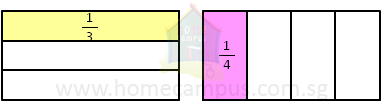But it is not clear which of the two fractions is greater. In order to compare the two fractions, we must first change them to like fractions. Here are the steps to change unlike fractions to like fractions:
Step 1:
Find the product of 3 and 4 (the two denominators).
3 × 4 = 12

Step 2:
Change each of the two fractions to its equivalent fraction so that the new denominator is 12.
 13 = ?12 => 1 × 43 × 4 = 412 14 = ?12 => 1 × 34 × 3 = 312

 Here's how to mark the two fractions on a single lasagna: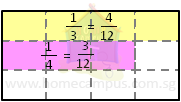Step 3:
 It is clear now that 412 , which is the equivalent of 13
 is greater than 312 which is the equivalent of 14 .
 Therefore, 13 is greater than 14 .

### Compare  16  and  14 .

Step 1:
Find the product of 6 and 4 = 24

Step 2:
Change each of the two unlike fractions to its equivalent so that the new denominator is 24.
 16 = ?24 => 1 × 46 × 4 = 424 14 = ?24 => 1 × 64 × 6 = 624

 Here's how the two fractions look like on a lasagna: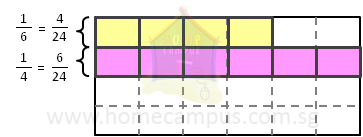Step 3:
 It is clear now that 624 or 14
 is greater than 424 or 16 .

### What is LCM or Least Common Multiple?

While the above method of converting unlike fractions to like fractions works, it is not the best method especially when dealing with more than two numbers or with bigger numbers. There is a better method to do so and it is called LCM or least common multiple.

Example:

To find out the multiples of a number, think of the multiplication table of that number.

The multiplication table of 6 is:
6 x 1 = 6, 6 x 2 = 12, 6 x 3 = 18, 6 x 4 = 24, 6 x 5 = 30, ...

Therefore, the multiples of 6 are:
6, 12, 18, 24, 30, ...
Find the LCM of 6 and 4.

Here are the steps to find the LCM of 6 and 4.
Step 1:
Find the multiples of 6 and the multiples of 4.
6 => 6, 12, 18, 24, 30, 36, 42, 48, 54, 60, 66, ...
4 => 4, 8, 12, 16, 20, 24, 28, 32, 36, 40, 44, ...

Step 2:
Find the common multiples of 6 and 4.
They are: 12, 24, 36, ...

Step 3:
Find the least (or smallest) of the common multiples of 6 and 4.
It is 12.
So, the LCM of 6 and 4 is 12.

### Compare 16 and 14 using LCM.

LCM or least common multiple, as we mentioned above, is a way to find out a new common denominator for the given unlike fractions so they can be changed to like fractions.

Here are the steps to compare the given unlike fractions:
Step 1:
Take the two unlike denominators, 6 and 4, and find a new common denominator using LCM.

Step 2:
We know from above that the LCM of 6 and 4 = 12.

Step 3:
Change the two unlike fractions so each has a denominator of 12.
 16 = ?12 => 1 × 26 × 2 = 212 14 = ?12 => 1 × 34 × 3 = 312

 This tells us we need not cut the lasagna into 24 pieces (6 × 4). It is sufficient enough to cut the lasagna into 12 pieces and still be able
 to mark the unlike fractions 16 and 14 on it for comparison.